×#### Thank you for registering.

One of our academic counsellors will contact you within 1 working day.

Click to Chat

1800-1023-196

+91-120-4616500

CART 0

• 0

MY CART (5)

Use Coupon: CART20 and get 20% off on all online Study Material

ITEM
DETAILS
MRP
DISCOUNT
FINAL PRICE
Total Price: Rs.

There are no items in this cart.
Continue Shopping• Complete JEE Main/Advanced Course and Test Series
• OFFERED PRICE: Rs. 15,900
• View Details

```Chapter 6: Algebraic Expressions and Identities Exercise – 6.2

Question: 1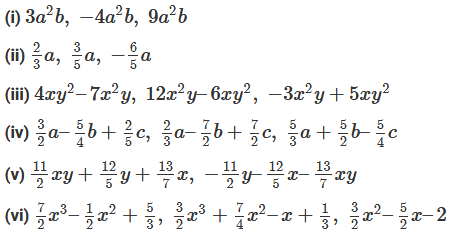Solution: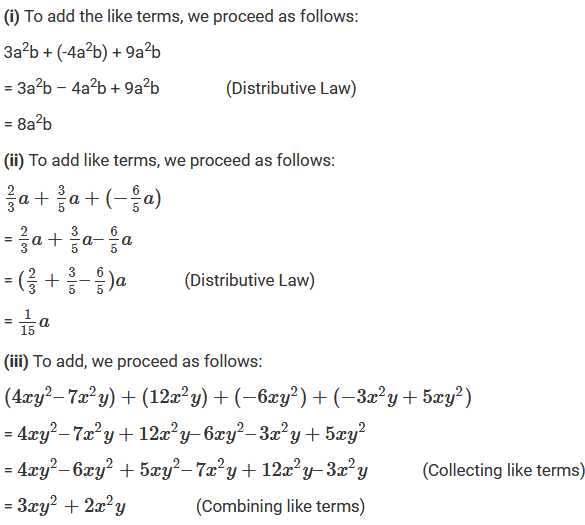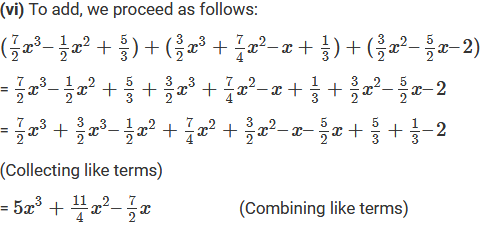Question: 2

Subtract:

(i) – 5xy from 12xy

(ii) 2a2 from -7a 2

(iii) 2a – b from 3a – 5bSolution:

(i) 12xy – (–5xy)

= 12xy + 5xy = 17xy

(ii) –7a2 – (2a2)

= –7a2 – 2a2 = –9a2

(iii) (3a – 5b) – (2a – b)

= (3a – 5b) – 2a + b

= 3a – 5b – 2a + b

= 3a – 2a – 5b + b = a – 4b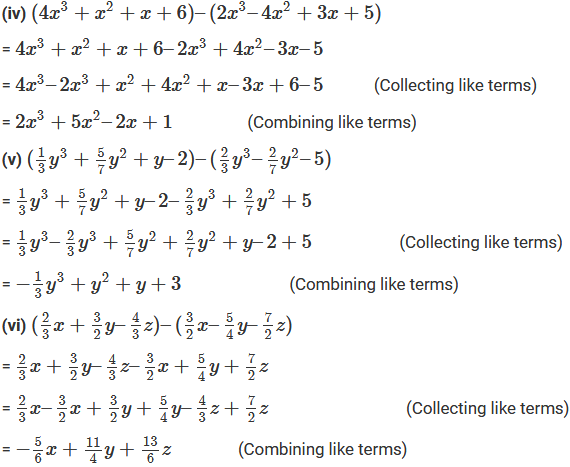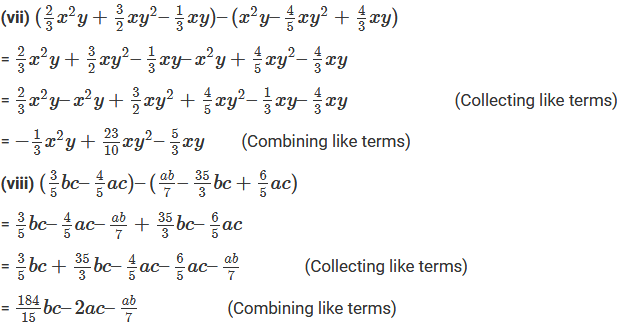Question: 3

Take away: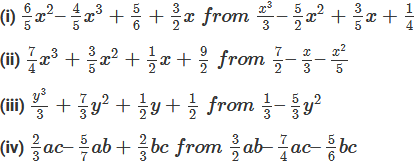Solution: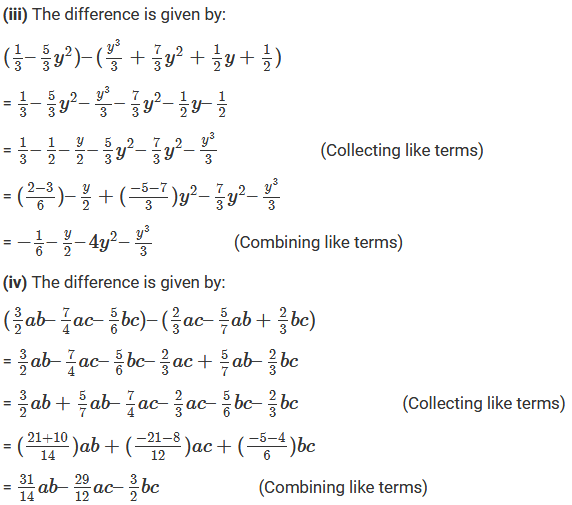Question: 4

Subtract 3x – 4y – 7z from the sum of x – 3y + 2z and –4x + 9y – 11z

Solution:

First add the expressions x – 3y + 2z and –4x + 9y – 11z we get:

(x – 3y + 2z ) + (–4x + 9y – 11z)

= x – 3y + 2z – 4x + 9y – 11z

= x – 4x – 3y + 9y + 2z – 11z      (Collecting like terms)

= –3x + 6y – 9z         (Combining like terms)

Now, Subtracting the expression 3x – 4y – 7z from the above sum, we get:

(–3x + 6y – 9z) – (3x – 4y – 7z)

= – 3x + 6y – 9z – 3x + 4y + 7z

= – 3x – 3x + 6y + 4y – 9z + 7z       (Collecting like terms)

= – 6x + 10y – 2z          (Combining like terms)

Thus, the answer is – 6x + 10y – 2z.

Question: 5

Subtract the sum of 3l – 4m – 7n2 and 2l + 3m – 4n2 from the sum of 9l + 2m – 3n2 and –3l + m + 4n2.

Solution:

We have to subtract the sum of (3l – 4m – 7n2) and (2l + 3m – 4n2) from the sum of (9l + 2m – 3n2) and (–3l + m + 4n2)

{(9l + 2m – 3n2) + (–3l + m + 4n2)} – {(3l – 4m – 7n2) + (2l + 3m – 4n2)}

= (9l – 3l + 2m + m – 3n2 + 4n2) – (3l + 2l – 4m + 3m – 7n2 – 4n2)

= (6l + 3m + n2) – (5l – m – 11n2)       (Combining like terms inside the parenthesis)

= 6l + 3m + n2 – 5l + m + 11n2

= 6l – 5l + 3m + m + n2 + 11n2      (Collecting like terms)

= l + 4m + 12n2       (Combining like terms)

Thus, the required solution is l + 4m + 12n2.

Question: 6

Subtract the sum 2x – x2 + 5 and –4x – 3 + 7x2 from 5.

Solution:

We have to subtract the sum of (2x – x2 + 5) and (–4x – 3 + 7x2) from 5.

5 – {(2x – x2 + 5) + (–4x – 3 + 7x2)}

= 5 – (2x – 4x – x2 + 7x2 + 5 – 3)

= 5 – 2x + 4x + x2 – 7x2 – 5 + 3

= 5 – 5 + 3 – 2x + 4x + x2 – 7x2       (Collecting like terms)

= 3 + 2x – 6x2    (Combining like terms)

Thus, the answer is 3 + 2x – 6x2.

Question: 7

Simplify each of the following: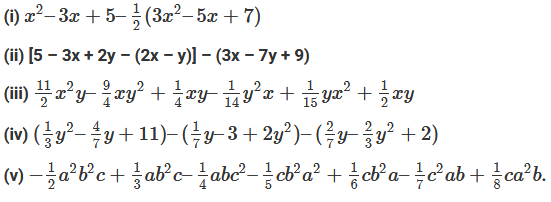Solution: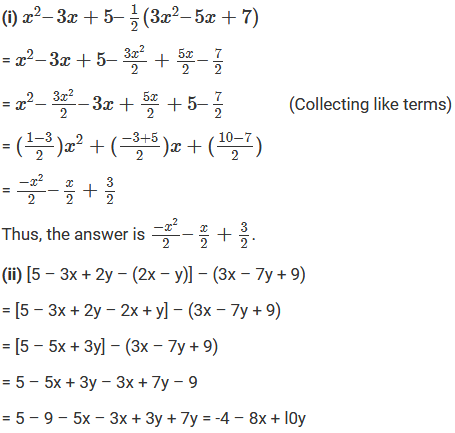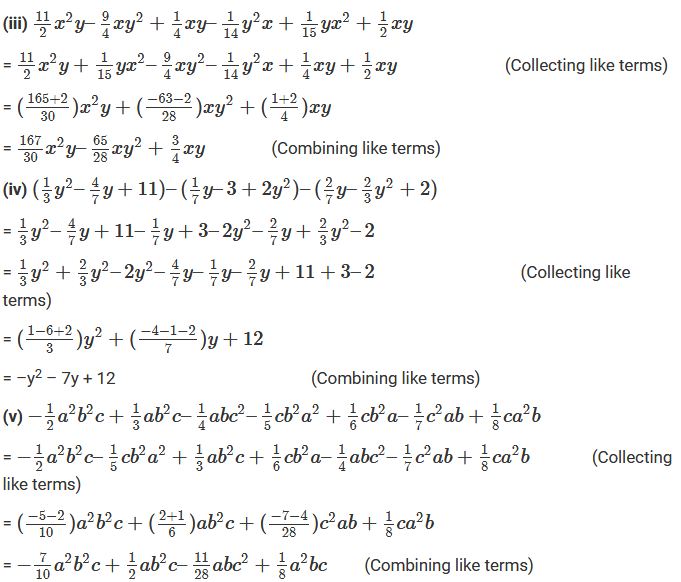```### Course Features

• 728 Video Lectures
• Revision Notes
• Previous Year Papers
• Mind Map
• Study Planner
• NCERT Solutions
• Discussion Forum
• Test paper with Video Solution